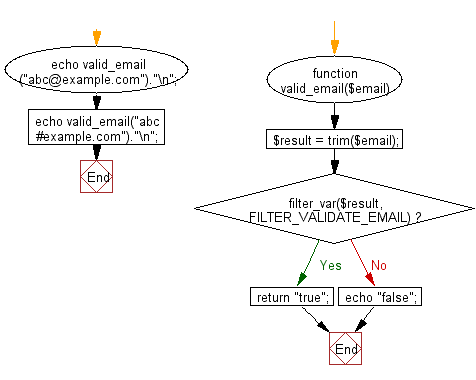﻿ PHP Exercise: Valid an email address - w3resource

# PHP Exercises: Valid an email address

## PHP: Exercise-38 with Solution

Write a PHP program to valid an email address.

Sample Solution: -

PHP Code:

``````<?php
function valid_email(\$email)
{
\$result = trim(\$email);
if (filter_var(\$result, FILTER_VALIDATE_EMAIL))
{
return "true";
}
else
{
echo "false";
}
}
echo valid_email("[email protected]")."\n";
echo valid_email("abc#example.com")."\n";
?>
```
```

Sample Output:

```true
false
```

Flowchart:PHP Code Editor:

Have another way to solve this solution? Contribute your code (and comments) through Disqus.

What is the difficulty level of this exercise?

Test your Programming skills with w3resource's quiz.

﻿

## PHP: Tips of the Day

Mutates the original array to filter out the values specified

Example:

```<?php
function tips_pull(&\$items, ...\$params)
{
\$items = array_values(array_diff(\$items, \$params));
return \$items;
}

\$items = ['x', 'y', 'z', 'x', 'y', 'z'];
print_r(tips_pull(\$items, 'y', 'z'));
?>
```

Output:

```Array
(
 => x
 => x
)
```